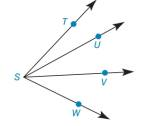Chapter 1.4, Problem 42E### Elementary Geometry for College St...

6th Edition
Daniel C. Alexander + 1 other
ISBN: 9781285195698

#### Solutions

Chapter
Section### Elementary Geometry for College St...

6th Edition
Daniel C. Alexander + 1 other
ISBN: 9781285195698
Textbook Problem
1 views

# If m ∠ T S V = 38 ° , m ∠ U S W = 40 ° , and m ∠ T S W = 61 ° , find m ∠ U S V .Exercises 44, 45

To determine

To find:

The measure of angle USV.

Explanation

Given:

mTSV=38°,mUSW=40° and mTSW=61°.

The given figure is,

Property used:

If a point say M lies in the interior of PQR, then mPQM+mRQM=mPQR.

Calculation:

The given figure is,

Figure (1)

Consider the figure (1),

U is the interior point of the angle TSW.

mUSW is 40° and mTSW is 61°.

Use above mentioned angle addition postulate to solve the measure of angle TSU.

### Still sussing out bartleby?

Check out a sample textbook solution.

See a sample solution

#### The Solution to Your Study Problems

Bartleby provides explanations to thousands of textbook problems written by our experts, many with advanced degrees!

Get Started

#### Find more solutions based on key concepts